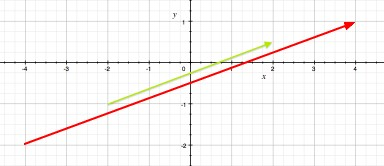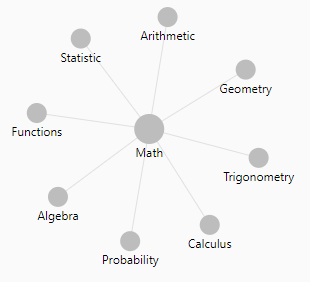# Number - Multiplication (Product)

Multiplication is denoted by:

• the cross symbol × (\times in Latex),
• by a point ·
• or by the absence of symbol

It's a binary function.

## Properties

Multiplication is:

• Associative: $\alpha(\beta.v) = (\alpha.\beta)v$

## Rules for handling the signed quantities

• Plus times plus makes plus
• Minus times minus makes plus
• Plus times minus makes minus
• Minus times plus makes minus.

## Documentation / Reference

Recommended Pages(Function|Operator) - Commutative property (Order doesn’t matter)

Commutative is an algebraic law. By commutative property , order in the operand doesn’t matter. Addition a + b = b + a Multiplication x . y = y . x commutativeFunction - Binary Function/Operation

A binary operation is an scalar operation with two arguments (arity of two) that produces one value. They are creating a binary relation. the addition operator, the multiplication operator ...Integer - Multiplication (Product)

Integer multiplication Input: 2 n digit numbers x and y where n is large in the thousands or even more Output: the product x times y Primitive Operation (unit of performance): add or multiply...Language - Expression

An Expression is a suite of special token that: are contained in statements and produce value (evaluate) They are a value specifications that specify values resulting from a computation. The expression...Linear Algebra - (Dot|Scalar|Inner) Product of two vectors

A dot Product is the multiplication of two two equal-length sequences of numbers (usually coordinate vectors) that produce a scalar (single number) Dot-product is also known as: scalar product or...Linear Algebra - Scalar (Multiplication|Product) - Scaling

Scalar Multiplication (Scaling) is the multiplication of a vector (for instance ) by a scalar (real number) (for instance ) to produce another vector (for instance ) Multiplying a vector v by a scalar...Mathematics - DistributiveMathematics - Inverse axiom

/ is inverse of - is inverse of + root is the inverse of exponentiation Functions f and g are functional inverses if: and are defined and are functions.Mathematics - homogeneity

MultiplicationNumber - (Arithmetical | Numerical | Mathematical) Operators

Number operators Addition (+) Addition in an compound assignment form += Subtraction (-) Division (/) Python: Multiplication () Truncating integer division (//) ...